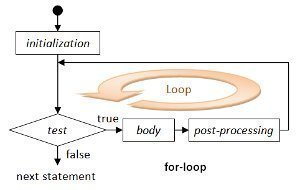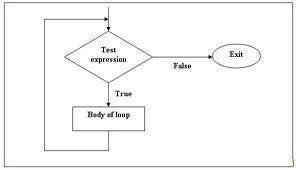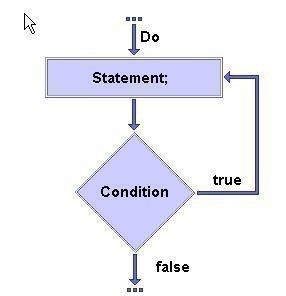•• # Java Loops – For, While, and Do

Java supports three types of loops: For, While, and Do.

## The Java For Loop

The Java for loop is a looping construct which continually executes a block of statements over range of values.

### Java for Loop Syntax

The syntax of a for loop in Java is:

```for (initialization; termination; increment) {
statement
}```

### Example Java for Loop

Here is a Java for loop which prints the numbers 1 through 10.

```for (int loopvar = 1; loopvar <= 10 ; loopvar++) {
System.out.println(loopvar);
}```

## The Java While Loop

A Java while loop is a looping construct which continually executes a block of statements while a condition remains true.

### Java while Loop Syntax

The syntax of a while loop in Java is:

``` while (expression) { statement } ```

### Example Java while Loop

Here is a Java while loop that prints the numbers 1 through 10.``

``` int loopvar = 1; // Declare and initialize the loop counter while (loopvar <= 10) { // Test and loop```
``` System.out.println(loopvar); // Print the variable loopvar = loopvar + 1; // Increment the loop counter } ```

## The Java Do Loop

A Java do loop is similar to the Java while loop, except that the while test happens at the bottom of the loop. This means that the loop always executes at least once.

### ``

The syntax of a do loop in Java is:

``` do { statement(s)```

` } while (expression); `

### Example Java do Loop

Here is a Java do loop that prints the number 1 — even though the test fails.

``` int loopvar = 1; // Declare and initialize the loop counter do { System.out.println(loopvar); // Print the variable loopvar = loopvar + 1; // Increment the loop counter } while (loopvar >= 10); // Test and loop ```

1. #### chaman

2 March, 2012 at 11:13 am

Thnks. Gud knwldge u hav. Helpful